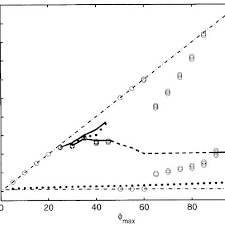## How to Calculate and Solve for Theoretical Value of g | Standard Formula | GravityThe image above represents theoretical value of g.

To compute for theoretical value of g, one essential parameter is needed and this parameter is Latitude (φ).

The formula for calculating theoretical value of g:

g = (-172 + 136sin²φ)

Where:

g = Theoretical Value of g | Standard Formula
φ = Latitude

Let’s solve an example;
Find the theoretical value of g when the latitude is 18.

This implies that;

φ = Latitude = 18

g = (-172 + 136sin²φ)
g = (-172 + 136sin²(18))
g = (-172 + 136(0.309)²)
g = (-172 + 136(0.0954))
g = (-172 + (12.986))
g = -159.01

Therefore, the theoretical value of g is -159.01 mGal.